This is the rendered collections of notes by me, Lex Toumbourou.

You can find the source on the GitHub project.

The notes are collecting using my interpretation of the Zettelkasten method.

More
• ## Cross product introduction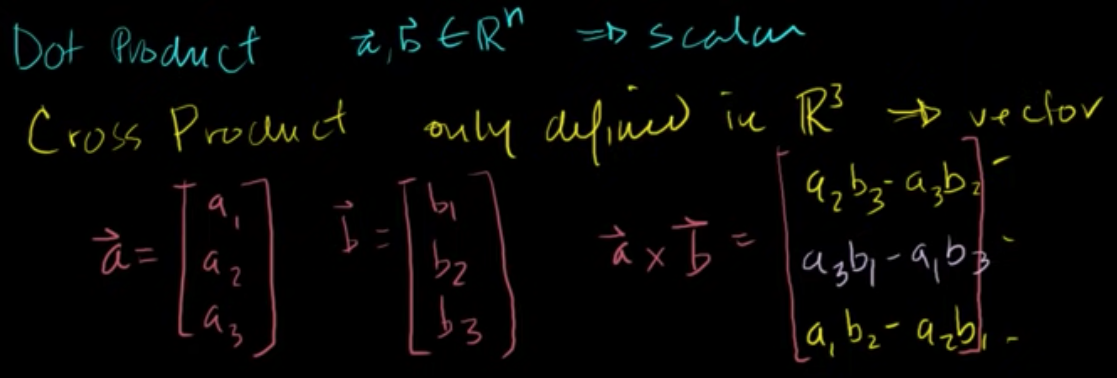Notes from Cross product introduction by Khan Academy.

• ## Matrix Determinate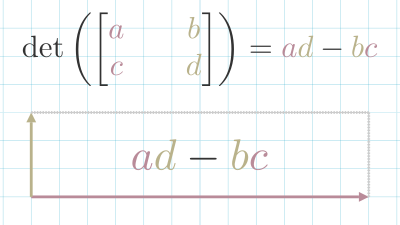A measure of how a matrix scales space.

• ## Matrix Transformation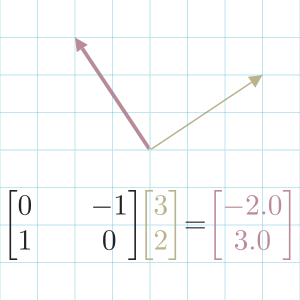A matrix as a transformation of a space.

• ## Basis Vectors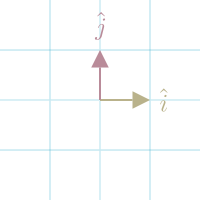The set of vectors that defines space.

• ## Roblox CFrame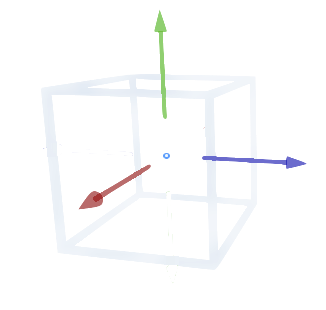In Roblox, a CFrame (coordinate frame) is an object that encodes position and rotation in 3D space.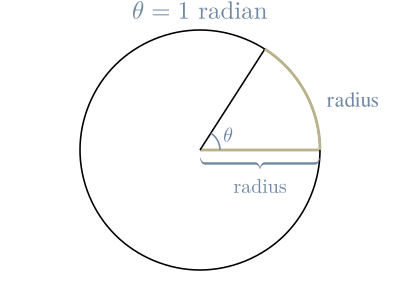A measurement of an angle in radiuses

• ## Matrix Inverse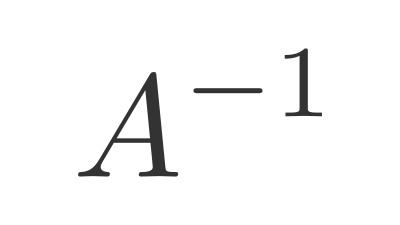A matrix that reverses a transformation.

• ## Identity Matrix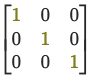When you multiply a matrix $(A)$ by the Identity Matrix $(I)$, you get the original matrix back.

$A …$

• ## Mathematics for Machine Learning

Notes from Coursera's Mathematics for Machine Learning: Linear Algebra by Imperial College London

• ## Law Of Cosines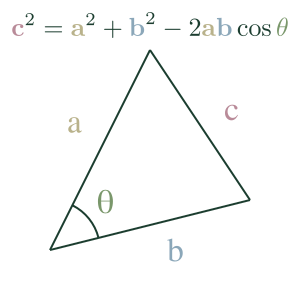The Law of Cosines expresses the relationship between the length of a triangle's sides and one of its angles.